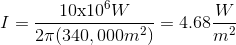# MCAT Physical : Intensity and Decibels

## Example Questions

### Example Question #1 : Intensity And Decibels

At a local concert, a speaker is set up to produce low-pitched base sounds with a frequency range of 20Hz to 200Hz, which can be modeled as  sine waves. In a simplified model, the sound waves the speaker produces can be modeled as a cylindrical pipe with one end closed that travel through the air at a velocity of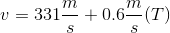, where T is the temperature in °C.

If the sound crew wanted to quadruple the intensity of the sound, they could __________.

halve the air density

double the amplitude of the sound wave

halve the amplitude of the sound wave

halve the frequency of the sound wave

double the amplitude of the sound wave

Explanation:

This question asks us to determine what would happen to the intensity of a sound wave if a variable could be changed. First, we need to remember the equation for intensity: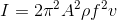, where A is the wave amplitude, rho is the density of the medium, f is the frequency of the wave, and v is the velocity of the wave.

This formula is important to understand qualitatively. For the MCAT, it is important to understand how the various factors impact the intensity of a wave. If we wanted to quadruple the intensity, we can see that we could double amplitude or double frequency. Seeing that we want the sound pitch to remain the same, we would want to double the amplitude and not the frequency. The relationship between intensity, frequency, and amplitude is important to understand.

### Example Question #2 : Intensity And Decibels

At a local concert, a speaker is set up to produce low-pitched base sounds with a frequency range of 20Hz to 200Hz, which can be modeled as  sine waves. In a simplified model, the sound waves the speaker produces can be modeled as a cylindrical pipe with one end closed that travel through the air at a velocity of, where T is the temperature in °C.

If the sound crew wanted to halve the intensity of the sound, they could __________.

double the amplitude of the sound wave

double the size of the room

double the frequency of the sound wave

halve the size of the room

double the size of the room

Explanation:

This question asks us to determine what would happen to the intensity of a sound wave if a variable could be changed. First, we need to remember the equation for intensity,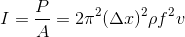, where P is the power of the wave, A is the area,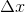is the wave amplitude, rho is the density of the medium, f is the frequency of the wave, and v is the velocity of the wave.

This formula is important to understand qualitatively. For the MCAT, it is important to understand how the various factors impact the intensity of a wave. If we wanted to halve the intensity, we can see that we could halve the power of the wave or double the area the wave is hitting; thus, we could double the size of the room, allowing the sound wave to have more room to spread out and increasing the area the wave interacts with.

### Example Question #3 : Intensity And Decibels

At a local concert, a speaker is set up to produce low-pitched base sounds with a frequency range of 20Hz to 200Hz, which can be modeled as  sine waves. In a simplified model, the sound waves the speaker produces can be modeled as a cylindrical pipe with one end closed that travel through the air at a velocity of, where T is the temperature in °C.

Find the intensity of a 35dB base wave produced by the speaker.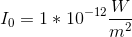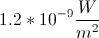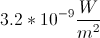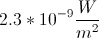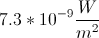Explanation:

This question asks us to determine the intensity of a wave, given to us as a comparison of the wave intensity to the intensity of the limit of human hearing. In math terms, we know that the decibel scale is calculated as shown below.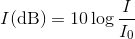I0 is the limit of human hearing (10-12 W/m2).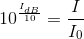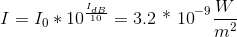### Example Question #4 : Intensity And Decibels

A song comes onto your car radio and you lower the volume from a setting ofto a setting of. How much has the intensity level decreased?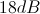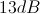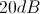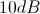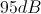Explanation:

Regardless of the units measured, a ten-fold decrease in volume equals a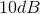decrease in intensity level.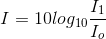Here, there is a twenty-fold decrease in sound intensity.

A ten-fold decrease would be achange, and a hundred-fold decrease would be a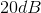change. A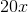decrease is closer to a ten-fold decrease than a hundred-fold decrease, hence the decrease in intensity level should be closer to.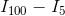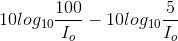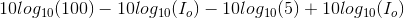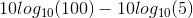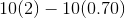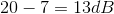### Example Question #1 : Intensity And Decibels

Which statement best explains why sound intensity is lessened when a wall is placed between the source and listener?

Part of the sound energy is reflected by the solid

Sound travels more slowly in solid than air

Sound frequency is lower in solid than air

Sound wavelength is shorter in solid than air

Part of the sound energy is reflected by the solid

Explanation:

When a sound wave contacts a surface, it transfers longitudinal vibration into the solid. Because of the increased density in the solid, these longitudinal compressions actually speed up and increase in wavelength. The frequency of the sound remains constant.

Only some of the sound energy is transferred to the solid, however. When the wave impacts the solid, some of the energy is bounced of the surface and reflected back to the source as an echo. This reduction of energy accounts for the lessened intensity experienced by a listener on the opposite side of the wall. Energy is also lost to internal reflection within the wall.

### Example Question #5 : Intensity And Decibels

You are watching a launching space shuttle that produces 10MW of sound from a horizantal distance of 300 meters away. After the shuttle has risen 500 meters straight up from its launch point, what intensity of sound do you percieve?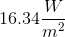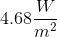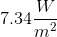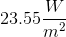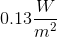Explanation:

As sound radiates from a point source, it does so in concentric circles. Therefore, we can calculate the intensity of sound using the following formula: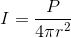We simply need to calculate: your distance from the space shuttle.

The shuttle is 500 meters from the ground, and you are 300 meters from its launch point. We can calculate the distance from you to the current position of the shuttle by using the Pythagorean Theorem: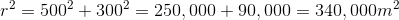There is no need to take the square root, since it's already in the form we need to do the calculation. Plugging in this value to the original equation, we get: